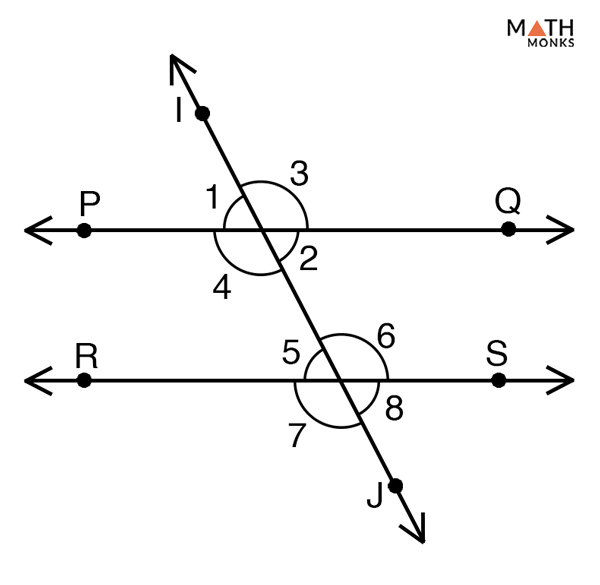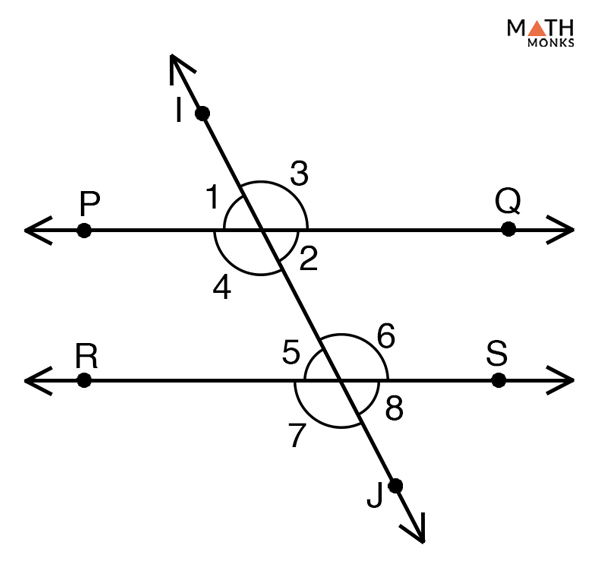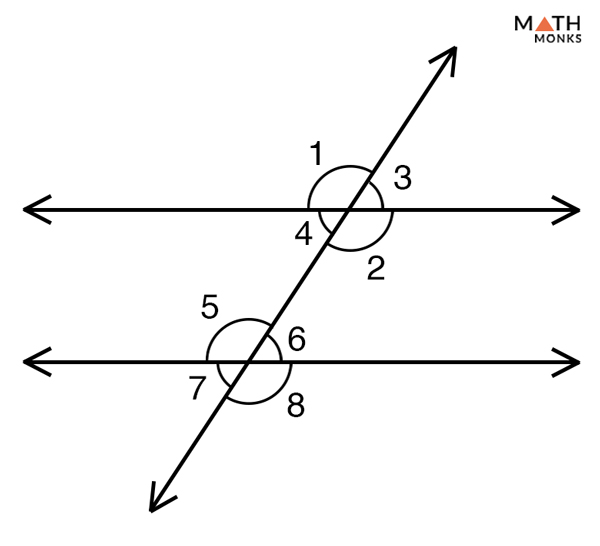# Corresponding Angles

## Definition

Corresponding angles are pairs of angles that occupy the same relative position at each intersection when a transversal intersects two parallel straight lines.

The above figure shows two parallel lines AB and CD intersected by the transversal GH. The pairs of corresponding angles in the given figure are:

∠1 and ∠5

∠3 and ∠6

∠4 and ∠7

∠2 and ∠8

Corresponding angles are equal if the transversal intersects at least two parallel lines.

In the given figure, AB∥CD,

Thus,

∠1 = ∠5, ∠3 = ∠6, ∠4 = ∠7, ∠2 = ∠8

Corresponding angles formed when a transversal intersects at least two non-parallel lines are not equal and are also found to have no relation with each other.

## Properties

1. Found on the same side of the transversal
2. Comprises of one interior and one exterior angle
3. They are equal if the transversal intersects two parallel lines
4. They are unrelated if the transversal intersects two non-parallel lines
5. They are supplementary if the transversal perpendicularly intersects two parallel lines.
6. Exterior angles on the same side of the transversal are supplementary if the two lines are parallel. This is same with interior angles.

## Types of Corresponding Angles

The two types of corresponding angles are:

1) Corresponding Interior Angle: Found at the inner side of the intersection between the parallel lines and the transversal.
2) Corresponding Exterior Angle: Found at the outer side of the intersection between the parallel lines and the transversal.

## Corresponding Angles TheoremProve Corresponding Angles Theorem

To prove:

∠1 = ∠5, ∠3 = ∠6, ∠4 = ∠7, ∠2 = ∠8

Proof:

Given, PQ and RS are the two parallel lines intersected by the transversal IJ.
Now, if PQ∥ RS
Then by corresponding angles theorem, we can write
∠1 = ∠5
∠3 = ∠6
∠4 = ∠7
∠2 = ∠8
Hence Proved
Remember: Thus, the only way to prove corresponding angles congruent is when the given lines are parallel. Thus, the Corresponding Angles Theorem is true without proof.

### Converse of Corresponding Angles TheoremProve the Converse of Corresponding Angles Theorem

To prove:

PQ∥ RS

Proof:

Given, ‘PQ’, ‘RS’ are two lines intersected by the transversal ‘IJ’, and ∠1 = ∠5, ∠3 = ∠6, ∠4 = ∠7, ∠2 = ∠8 are the pairs of corresponding angles
Then, by the Converse of Interior Angles Theorem,
PQ∥ RS
Hence Proved
Remember: Thus, the only way to prove the given lines parallel is just by stating the corresponding angles congruent. Thus the Converse of Corresponding Angles Theorem is accepted as true without proof.

## How to Find Corresponding Angles

Let us take some examples to understand the concept better.Find the missing angles in the given diagram. Given that ∠3 = 45°

Solution:

Given, ∠3 = 45°
∠3 = ∠4 (Vertically opposite angles)
Hence, ∠4 = 45°
Now,
∠4 =∠7 = 45° (Corresponding Angles)
∠3 =∠6 (Corresponding Angles)
Hence, ∠6 = 45°
Now,
∠1 + ∠4 = 180° (Supplementary angles)
∠1 + 45° = 180°
∠1 = 180° – 45°
∠1 =135°
Similarly,
∠1 = ∠2 (Vertically opposite angles)
Hence, ∠2 = 135°
Again,
∠1 = ∠5 (Corresponding Angles)
Hence, ∠5 = 135°
Similarly,
∠2 = ∠8 (Corresponding Angles)
Hence, ∠8 = 135°

The two corresponding angles measure (2x +10)° and 70°. Find the value of x.

Solution:

According to the corresponding angles theorem, the two corresponding angles are congruent.
Thus,
(2x +10)° = 70°
2x = 70° – 10°
x = 60°/2
x = 30°

The two corresponding angles of the given figure is 6y-14 and 4y + 6. Find the magnitude of a corresponding angle.

Solution:

According to the corresponding angles theorem, the two corresponding angles are congruent.
Thus,
6y-14 = 4y + 6
6y – 4y = 6 + 14
2y = 20
y = 10
Thus, the magnitude is,
6y-14 = 6 x 10 – 14 = 46°

## Examples in Real Life

• Windows have horizontal and vertical grills, which make multiple squares. Each of the vertex makes corresponding angles
• A bridge standing on a pillar, where each pillars are connected to each other in such a way that corresponding angles are equal
• The design of the railway track where the corresponding angles are kept equal
• Angles formed in a Rubik’s cube

## FAQs

Q1. Are corresponding angles supplementary

Ans. Corresponding angles are supplementary only if the transversal perpendicularly intersects two parallel lines.